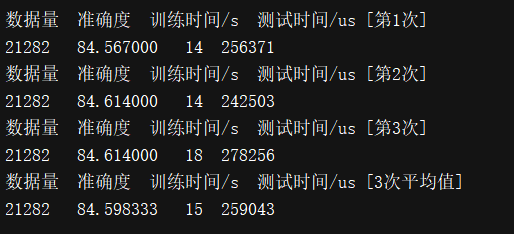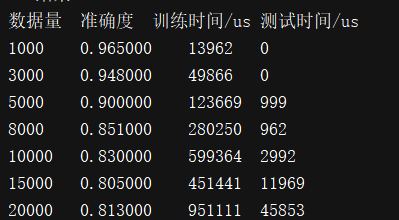# 2、Liblinear中的效果和Sklearn中的效果对比

liblinear中的支持向量机(SVM)算法：liblinear的具体使用方法可以参考我的这篇文章：机器学习 之 liblinear的帮助文档翻译

# 3、Liblinear实验整体流程

1、先把整体样本的文件转换成liblinear可读形式的文件

3、把整体向量按照8：2的比例，分为80%的训练集，20%的测试集

4、接着把训练集的向量和标签统一的传入Liblinear算法的train函数中，得到拟合后的模型

5、然后，通过predict函数把测试向量、标签和模型丢进去

6、最后通过evaluations把结果输出

7、最后做成表格形式以便观看

8、循环多次，求平均值

# 4、核心源码

import jieba
import datetime
# 向量\测试集\训练集\得分比对
from sklearn.model_selection  import train_test_split
from sklearn.feature_extraction.text import TfidfTransformer
from sklearn.feature_extraction.text import CountVectorizer

from converter import AnneTextConverter
# 支持向量机
from liblinearutil import *

# 忽略警告
import warnings
warnings.filterwarnings("ignore", category=FutureWarning, module="sklearn", lineno=196)

# all
m_list_allText = []
m_list_allL4ID = []
# 内容的训练集、测试集
m_text_test = []
m_text_train = []
m_label_test = []
m_label_train = []

m_list_all = []
m_map_all = {}
m_test_map = {}

numCount = "21282"
# 测试
def test(count):
# 生成字典
anne = AnneTextConverter()

anne.convert_text(text_src="rg_train_20190102_20181227114134.train",  delimiter=":", output="rg_train_20190102_20181227114134.svm",labelLevel="L4")
# 随机分为 测试集 和 训练集 2-8分
m_text_train, m_text_test, m_label_train, m_label_test = train_test_split(m_list_allText, m_list_allL4ID, test_size=0.2, random_state=0)

# 数据大小
print("总集大小:", count)

# 训练
startT_Train = datetime.datetime.now()

# -q : 安静模式（无输出信息）
# print("m_label_train",m_label_train)
# print("m_text_train",m_text_train)
model = train(m_label_train, m_text_train, "-s 4 -q")

save_model("liblinear.svm",model)
endT_Train = datetime.datetime.now()
print("训练时间Time:%ds"%(endT_Train - startT_Train).seconds)

# 测试
startT = datetime.datetime.now()
pLabel,pAcc,pVal = predict(m_label_test, m_text_test, model)
endT = datetime.datetime.now()
print("测试Time:", (endT - startT).microseconds)

ACC,MSE,SCC = evaluations(m_label_test,pLabel)

# 计算百分比
print("准确率:", round(ACC, 3))

global m_map_all
m_map_all["精确率"]=round(ACC, 3)
m_map_all["数据量"]=count
m_map_all["训练时间/s"]=(endT_Train - startT_Train).seconds
m_map_all["测试时间/us"]=(endT - startT).microseconds
print("m_map_all:",m_map_all)

def runOnce(i):
global m_map_all
print ("-- 开始 --")
test(numCount)
print ("-- 结束 --")

# 打印表格
print("数据量\t准确度\t训练时间/s\t测试时间/us")
print("%s\t%f\t%d\t%d"%(m_map_all["数据量"],m_map_all["精确率"],m_map_all["训练时间/s"],m_map_all["测试时间/us"]))
m_test_map[i] = m_map_all
m_map_all = {}

if __name__ =="__main__":
# 跑n次
runCount = 3
for i in range(runCount):
runOnce(i)

# 打印n次的结果
for i in range(runCount):
tmp = m_test_map[i]
# 打印表格
print("数据量\t准确度\t训练时间/s\t测试时间/us [第%d次]"%(i+1))
print("%s\t%f\t%d\t%d"%(tmp["数据量"],tmp["精确率"],tmp["训练时间/s"],tmp["测试时间/us"]))

# n次平均值
print("数据量\t准确度\t训练时间/s\t测试时间/us [%d次平均值]"%(runCount))
tmpMap = m_test_map
# tmpList = tmpMap
tmpCount = tmpMap["数据量"]
tmpScore = 0
tmpTrainTime = 0
tmpTestTime = 0
for i in range(runCount):
tmpScore += m_test_map[i]["精确率"]
tmpTrainTime += m_test_map[i]["训练时间/s"]
tmpTestTime += m_test_map[i]["测试时间/us"]
print("%s\t%f\t%d\t%d"%(tmpMap["数据量"],tmpScore/runCount,tmpTrainTime/runCount,tmpTestTime/runCount))



# 5、其它相关文章

04-021800

#### 【Python】Mac Python安装liblinear机器学习库

02-202828

#### 机器学习 之 sklearn中的支持向量机（SupportVectorMachine）文本算法的精确率07-17446

#### 如何解决TextGrocery的python3兼容问题

02-218366

#### 机器学习 之 liblinear的帮助文档翻译

07-02763

#### 海量数据分类 liblinear使用总结

09-0326

#### LibLinear（SVM包）使用说明之（三）实践

01-1597

#### sklearn中的SVM模型

02-1759

#### Tgrocery学习及使用

09-026738

#### （一）由源码学算法：通过scikit-learn库代码示例学机器学习算法（总）

10-223518

#### Liblinear使用

09-027万+

08-063871

#### TextGrocery短文本分类使用

07-177664

#### 在python中使用liblinear

01-311万+

#### 系统学习机器学习之SVM(三)--Liblinear,LibSVM使用整理，总结

02-27566

#### PCA降维后出现准确率低的问题的一种解决方法经验

09-301191

#### Libsvm(liblinear)工具箱的下载，安装及使用

02-215416

#### mac osx libsvm与python接口:ModuleNotFoundError: No module named 'svm'

07-314555

#### ModuleNotFoundError: No module named 'scipy._lib.decorator'问题解决©️2020 CSDN 皮肤主题: 代码科技 设计师: Amelia_0503点击重新获取扫码支付1.余额是钱包充值的虚拟货币，按照1:1的比例进行支付金额的抵扣。
2.余额无法直接购买下载，可以购买VIP、C币套餐、付费专栏及课程。余额充值